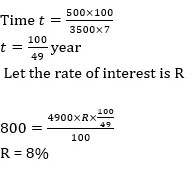# Quant Quiz for SBI PO PRE & LIC AAO 2019 |27th April 2019

## Quant Quiz for SBI PO PRE & LIC AAO 2019

Reasoning Quiz to improve your Quantitative Aptitude for SBI Po & SBI clerk exam Reasoning, IBPS PO Reasoning , IBPS Clerk Reasoning , IBPS RRB Reasoning, LIC AAO ,  and other competitive exams.

Q1. A & B working together can complete a piece of work in 72 days, B & C working together can complete the same work in 90 days and A & C working together can complete the same work in 75 days. If A, B & C starts working together, then find in how many days will the same work be completed?

A)B)C)D)E)Q1. Ans(B)Q2.  Shubham work for 5 days and remaining work was completed by Harvinder in 9 days. If Harvinder work for 12 days then remaining work was completed by Shubham in 3 days, then find how much time Harvinder will take to complete the work alone.

A)B)C)D)E)Q2. Ans(B)Q3.  Speed of a boat in still water and speed of current is in ratio 6 : 1. If the difference between distance covered by boat in 2 hours upstream and in 2 hours downstream is 8 km.
Quantity-1- Speed of boat in still water
Quantity-2- speed of cyclist who goes 28 km in 2 hrs.

(A) Quantity I > Quantity II

(B) Quantity I < Quantity II

(c) Quantity I ≥ Quantity II

(D) Quantity I ≤ Quantity II

(E) Quantity I = Quantity II or no relation

Q3. Ans(B)Q4. Quantity I — cost price of an article having marked price = Rs. 400, which when sold at 20% discount still make a gain ofQuantity II — cost price of an article which is sold at 14% profit and if cost price and selling price both are Rs. 117 less, the profit would be 9% more.

(A) Quantity I > Quantity II

(B) Quantity I < Quantity II

(c) Quantity I ≥ Quantity II

(D) Quantity I ≤ Quantity II

(E) Quantity I = Quantity II or no relation

Q4. Ans(A)Q5.  Quantity I — the sum of money for which the difference between SI and CI obtained on it in 2 years at 6% per annum compounded annually is Rs. 43.2.
Quantity II — Rs. 12850

(A) Quantity I > Quantity II

(B) Quantity I < Quantity II

(c) Quantity I ≥ Quantity II

(D) Quantity I ≤ Quantity II

(E) Quantity I = Quantity II or no relation

Q5. Ans(B)Q6. A man borrows Rs. 4000 at 20% compound rate of interest. At the end of each year he pays back Rs. 1500. How much amount should he pay at the end of the third year to clear all his dues ?

(a) Rs. 2592

(b) Rs. 2852

(c) Rs. 2952

(d) Rs. 2953

(e) None of these

Q6. Ans(c)[/su_spoiler]

Q7. Ashok borrowed some money at simple interest at the rate of 6 per cent per annul for the first two years, at the rate of 9 per cent per annul for the next three years and at the rate of 14% per cent per annul for the period beyond five years. If he pays a total interest of Rs. 11400 at the end of 9 years how much money did he borrow ?

(a) Rs. 16,000

(b) Rs. 14,000

(c) Rs. 18,000

(d) Rs. 12,000

(e) None of these

Q7. Ans(d)Q8. On Rs 3500 invested at a simple interest rate 7 per cent per annum, Rs 500 is obtained as interest in certain years, In order to earn Rs 800 as interest on Rs 4900 in the same number of years, what should be the rate of simple interest?

(a) 9%

(b) 10%

(c) 12%

(d) 8%

(e) None of these

Q8. Ans(d)10.  A takes half as long to do a piece of work as B takes, and C does it in the same time as A and B together, and if all three working together would take 7 days, how long would each take separately?

A)B)C)D)E)Q10. Ans(A)### You Can Read This Also:

Best E-books for LIC AAO 2019 : Get PDF here

ambitiousbaba.com need your support to Grow

I challenge you will get Best Content in Our PDFs with Detail solutions and Latest Pattern

Memory Based Puzzle E-book | 2016-19 Exams Covered

Get PDF here

Caselet Data Interpretation 200 Questions

Get PDF here
Puzzle & Seating Arrangement E-Book for BANK PO MAINS (Vol-1)

Get PDF here

### ARITHMETIC DATA INTERPRETATION 2019 E-book

Get PDF here
The Banking Awareness 500 MCQs E-book| Bilingual (Hindi + English)

Get PDF here

High Level DATA INTERPRETATION Practice E-BOOK

Get PDF here

3